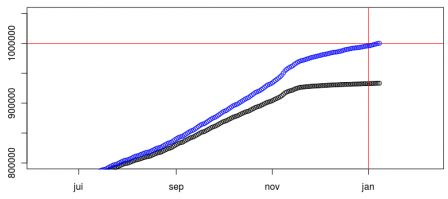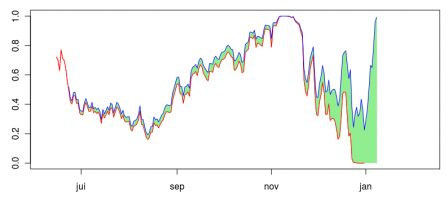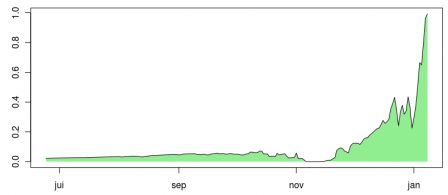Want to share your content on R-bloggers? click here if you have a blog, or here if you don't.

Just after arriving in Montréal, at the beginning of September, I discussed statistics of my blog, and said that it might be possible – or likely – that by new year’s Eve, over a million page would have been viewed on my blog (from Google’s counter, here). By the end of October (here) I was very optimistic, but mi-December (here) the challenge was likely to be failed. An indeed, the million page target was hit one week after, on January 8th,
`base=read.table("http://freakonometrics.blog.free.fr/public/data/million1.csv",sep="t",header=TRUE)X1=cumsum(base\$nombre)X0=X1base=read.table("http://freakonometrics.blog.free.fr/public/data/million2.csv",sep="t",header=TRUE)X2=cumsum(base\$nombre)X=X1+X2 D0=as.Date("08/11/2008","%d/%m/%Y")D=D0+1:length(X1)plot(D,X1,xlim=c(as.Date("08/06/2010","%d/%m/%Y"),as.Date("08/02/2011","%d/%m/%Y")),ylim=c(800000,1050000))abline(h=1000000,col="red")abline(v=as.Date("01/01/2011","%d/%m/%Y"),col="red")points(D,X,col="blue")`Again, the black points were from the previous blog (http://blogperso.univ-rennes1.fr/arthur.charpentier/) which was transferred to that new one (http://freakonometrics.blog.free.fr) this Autumn. So I just sum up the stats to get the blue points. At each date, I fit an ARIMA, and use it to make forecast the total number of pages viewed on January 1st, and calculate the probability to reach a million page viewed at that date (using a Gaussian ARIMA model). Actually, here, I changed a little bit the challenge, and asked “what would have been the probability to reach a million page viewed on January 1st, and on January 8th” ?

`kt=which(D==as.Date("01/06/2010","%d/%m/%Y"))Xbase=XX=X1+X2P1=P2=rep(NA,(length(X)-kt)+7)for(h in 0:(length(X)-kt+7)){model  <- arima(X[1:(kt+h)],c(7 ,1,7),method="CSS")forecast <- predict(model,200) u=max(D[1:kt+h])+1:300if(min(u)<=as.Date("01/01/2011","%d/%m/%Y")){k=which(u==as.Date("01/01/2011","%d/%m/%Y"))(P1[h+1]=1-pnorm(1000000,forecast\$pred[k],forecast\$se[k]))}k=which(u==as.Date("08/01/2011","%d/%m/%Y"))(P2[h+1]=1-pnorm(1000000,forecast\$pred[k],forecast\$se[k]))}`
The red curve is the probability to reach 1 million viewed on January 1st (as done earlier, using an ARIMA projection). The blue one is the probability to reach 1 million viewed one week after, on January 8th.and here is the difference between probabilities,The flat part at the beginning of November corresponds to the bump that was observed on the initial graph. But then, the slope was too low, and in December, the challenge was failed... Obviously, looking at statistics during a blog migration is not a bright idea...